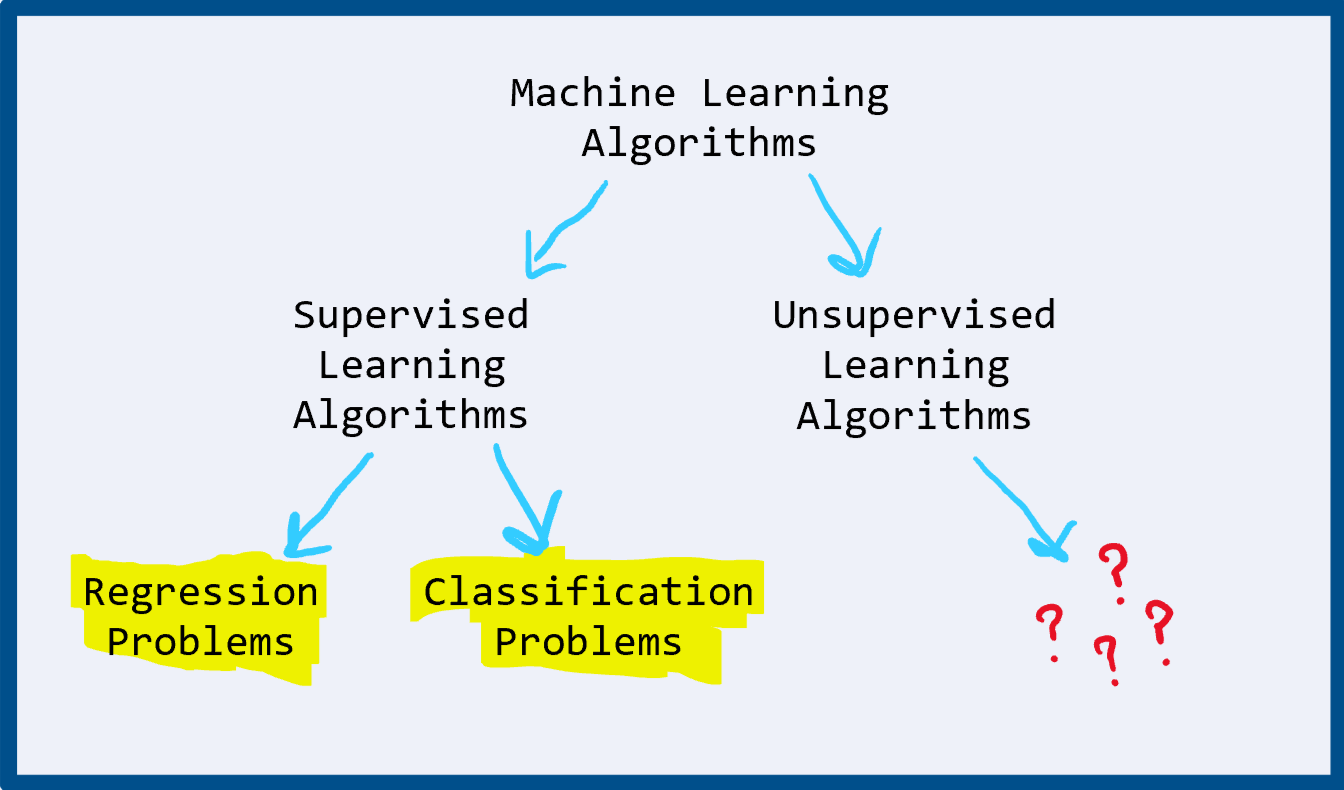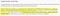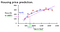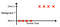# Machine Learning Basics: Linear Regression Vs Logistic Regression in 5 minutesML Algorithms Overview

So a few months ago I decided to take the highly-regarded Intro to Machine Learning course taught by Andrew Ng on coursera. It’s an 11-week introduction to core concepts in machine learning and, overall, a great place to start your machine learning journey - no matter who you ask. Wherever you are in that journey, knowing the difference between linear and logistic regression is very necessary. In fact, I encountered this question in an interview just last week and I certainly could have answered it better. Don’t be like me, get it right before the interview.Andrew Ng, Intro to Machine Learning

The first thing to understand is that supervised learning algorithms are used whenever we understand what the answer (output) should look like before training our model. For example, predicting housing prices using only the square footage as a clue. It is possible to gather a dataset of housing prices and their associated square footages to train our model on, therefore we can use a supervised learning algorithm. If the task was instead to drive a car, we cannot easily create a dataset to train a model how to do this. Instead we would have to use an unsupervised learning algorithm, but let’s not worry about that for now.

# Regression: Linear and Logistic

Linear regression is essentially training a model to draw a line through a set of points. The closer to each and every point the line becomes, the more accurate the model. This is a bit of an oversimplification, but not by much! Take the example of predicting housing prices using square-footages as a clue:The red X’s represent a single house and the lines are generated using linear regression

Input: square footage

Output: housing price

The lines above represent the output of linear regression: a function where the input is square footage and the output is housing price. Using this function, you can accurately predict housing prices for houses the model has not yet seen. After all, if the algorithm could only predict the price of houses it has seen before, it wouldn’t be very useful, right?

Logistic regression is similar, but not the same. In logistic regression the function isn’t linear, it’s logistic. This means our output is between 0 and 1 and is usually interpreted as a probability (by convention). Take the example of detecting cancer in patients. Instead of square footage, we will use tumor size as our clue (variable). Our dataset would contain tumor sizes and whether or not the tumors were malignant (represented by a 0 or a 1).Dataset for determining cancer in patients

Input: tumor size

Output: probability of malignant tumor

Now, at this point you might be thinking “This looks a lot like classification, I thought we were talking about regression” and you’re right. Logistic regression can be taken one step further to solve a classification problem (and it usually is!). If we decide that a probability greater than 0.5 represents a malignant tumor and anything less represents a benign tumor, then voila! We have created discrete categories from our continuous input, AKA, classification. I know it seems like semantics, but it’s important to know the difference!

# So, in summary:

Regression is used to map input variables to some continuous function, be it linear or logistic, while classification is used to map input variables to discrete categories.

Logistic Regression can be used to solve classification problems, but it is still regression.

I hope you enjoyed this explanation. Have you also taken Andrew Ng’s Machine Learning course? Are you considering it? Where are you in your machine learning journey?

Leave a comment and let me know!

Big time nerd